+

# Fractional Exponents and Radicals

##### Rating:
(2)
• (1)
• (0)
• (0)
• (0)
• (1)
Author: Sophia Tutorial
##### Description:

Write fractional exponents as radicals, or vice versa.

(more)
Tutorial
what's covered
1. Inverse Operations: Radicals and Exponents
2. Fractional Exponents and Radicals

# 1. Inverse Operations: Radicals and Exponents

Just as multiplication and division are inverse operations of one another, radicals and exponents are also inverse operations. For example, suppose we have the the number 3 and we raise it to the second power. Now if we were to take the square root of 32, notice that we will end up with 3. This is the number with which we started.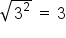Below are a few more examples: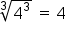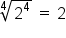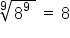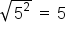# 2. Fractional Exponents and Radicals

Whenever we are working with the exponents, taking the appropriate radical will always cancel out the exponent operation. That is to say, if a number is raised to the power of a, we can cancel out the exponent by taking the resulting value and taking the a-th root of the result. This is the same as raising the the resulting number to a fractional exponent. For example, if we have 74, in order to cancel out the exponent 4 we would have to take the 4th root of 74 which is the same as raising 74 to the ¼ power. This is shown below:In general, whenever we have an expression raised to the a-th power, we can take the a-th root of that number, which is the same as raising that number to the 1/a power. By doing this, we effectively cancel out the radical with the exponent.Let’s look at this a little more in-depth. When we simplify radicals with exponents, we divide the exponent by the index. Another way to write division is with a fraction bar. This is how we will define rational exponents.

formula
Property of Fractional Exponents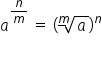The denominator of a rational exponent becomes the index on our radical. Likewise, the index on the radical becomes the denominator of the exponent. We can use this property to change any radical expression into an exponential expression.Index is denominatorNegative exponents from reciprocals

We can also change any rational exponent into a radical expression by using the denominator as the index.Index is denominatorNegative exponent means reciprocals

The ability to change between exponential expressions and radical expressions allows us to evaluate problems we had no means of evaluating before. We do this by changing the expression to a radical.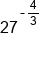Change to radical, denominator is index, negative means reciprocal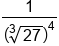Evaluate radical

summary
Exponents and radicals are inverse operations of each other, meaning they cancel each other out. Fractional exponents and radicals can be written as one another by using the property of fractional exponents. The largest advantage of being able to change a radical expression into an exponential expression is that we are now allowed to use all of our exponent properties to simplify.

Source: Adapted from "Beginning and Intermediate Algebra" by Tyler Wallace, an open source textbook available at: http://wallace.ccfaculty.org/book/book.html

Formulas to Know
Property of Fractional ExponentsRating Header